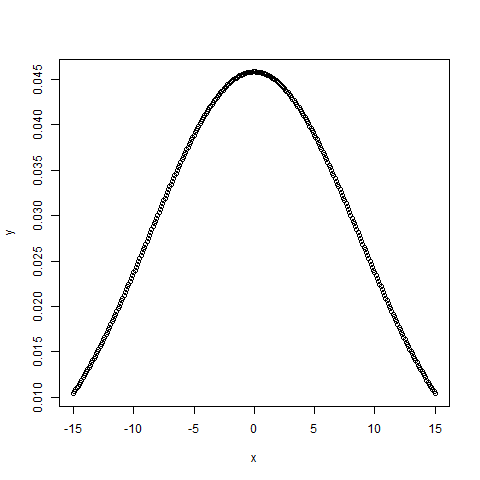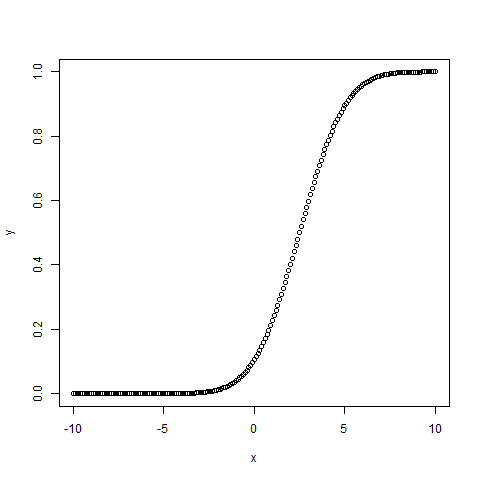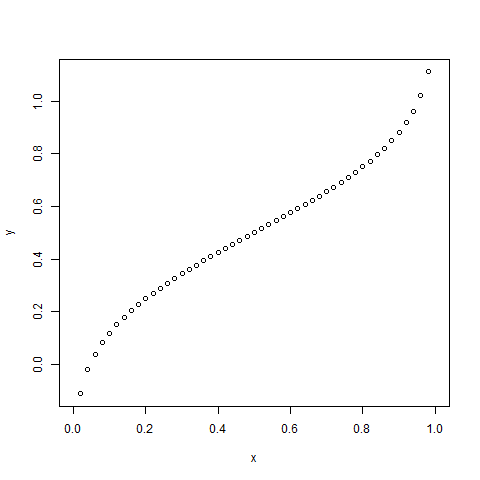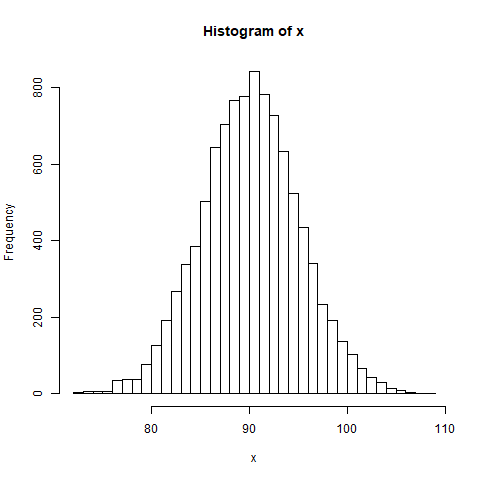# Normal Distribution in R

Normal Distribution is a probability function used in statistics that tells about how the data values are distributed. It is the most important probability distribution function used in statistics because of its advantages in real case scenarios. For example, the height of the population, shoe size, IQ level, rolling a dice, and many more.

It is generally observed that data distribution is normal when there is a random collection of data from independent sources. The graph produced after plotting the value of the variable on x-axis and count of the value on y-axis is bell-shaped curve graph. The graph signifies that the peak point is the mean of the data set and half of the values of data set lie on the left side of the mean and other half lies on the right part of the mean telling about the distribution of the values. The graph is symmetric distribution.

In R, there are 4 built-in functions to generate normal distribution:

• dnorm()
dnorm(x, mean, sd)
• pnorm()
pnorm(x, mean, sd)
• qnorm()
qnorm(p, mean, sd)
• rnorm()
rnorm(n, mean, sd)

where,

x represents the data set of values
mean(x) represents the mean of data set x. It’s default value is 0.sd(x) represents the standard deviation of data set x. It’s default value is 1.n is the number of observations.
p is vector of probabilities

### Functions To Generate Normal Distribution in R

#### dnorm()

dnorm() function in R programming measures density function of distribution. In statistics, it is measured by below formula-where,is mean andis standard deviation.

Syntax :

dnorm(x, mean, sd)

Example:

 # creating a sequence of values   # between -15 to 15 with a difference of 0.1  x = seq(-15, 15, by=0.1)      y = dnorm(x, mean(x), sd(x))      # output to be present as PNG file  png(file="dnormExample.png")      # Plot the graph.  plot(x, y)      # saving the file  dev.off()

Output:#### pnorm()

pnorm() function is the cumulative distribution function which measures the probability that a random number X takes a value less than or equal to x i.e., in statistics it is given by-Syntax:

pnorm(x, mean, sd)

Example:

 # creating a sequence of values  # between -10 to 10 with a difference of 0.1  x <- seq(-10, 10, by=0.1)     y <- pnorm(x, mean = 2.5, sd = 2)     # output to be present as PNG file  png(file="pnormExample.png")     # Plot the graph.  plot(x, y)     # saving the file  dev.off()

Output :#### qnorm()

qnorm() function is the inverse of pnorm() function. It takes the probability value and gives output which corresponds to the probability value. It is useful in finding the percentiles of a normal distribution.

Syntax:

qnorm(p, mean, sd)

Example:

 # Create a sequence of probability values   # incrementing by 0.02.  x <- seq(0, 1, by = 0.02)     y <- qnorm(x, mean(x), sd(x))     # output to be present as PNG file  png(file = "qnormExample.png")     # Plot the graph.  plot(x, y)     # Save the file.  dev.off()

Output:#### rnorm()

rnorm() function in R programming is used to generate a vector of random numbers which are normally distributed.

Syntax:

rnorm(x, mean, sd)

Example:

 # Create a vector of 1000 random numbers  # with mean=90 and sd=5  x <- rnorm(10000, mean=90, sd=5)     # output to be present as PNG file  png(file = "rnormExample.png")     # Create the histogram with 50 bars  hist(x, breaks=50)     # Save the file.  dev.off()

Output :My Personal Notes arrow_drop_upBlockchain Enthusiast

If you like GeeksforGeeks and would like to contribute, you can also write an article using contribute.geeksforgeeks.org or mail your article to contribute@geeksforgeeks.org. See your article appearing on the GeeksforGeeks main page and help other Geeks.

Please Improve this article if you find anything incorrect by clicking on the "Improve Article" button below.

Article Tags :

3

Please write to us at contribute@geeksforgeeks.org to report any issue with the above content.